Saturday, December 4, 2021
Home > CBSE Class 10 > Statistics Mean Median Mode CBSE Class 10 MCQ Questions with Answers Keys

# Statistics Mean Median Mode CBSE Class 10 MCQ Questions with Answers Keys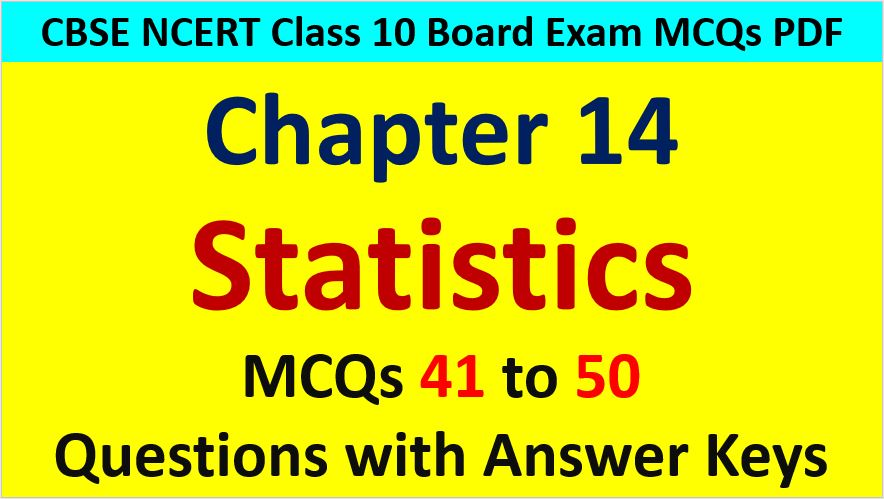Hi students, Welcome to AMBiPi (Amans Maths Blogs). In this article, you will get Statistics Mean Median Mode CBSE Class 10 MCQ Questions with Answer Keys. You can download this PDF and save it in your mobile device or laptop etc.

Statistics Class 10 MCQ Question No 41:

If the mean of the observation x, x + 3, x + 5, x + 7 and x + 10 is 9, the mean of the last three observation is

Option A : 10 1/3

Option B : 10 2/3

Option C : 11 1/3

Option D : 11 2/3

Option C : 11 1/3

Statistics Class 10 MCQ Question No 42:

If the mean of the numbers 27 + x, 31 + x, 89 + x, 107 + x, 156 + x is 82, then find the mean of the numbers 130 + x, 126 + x, 68 + x, 50 + x, 1 + x is

Option A : 75

Option B : 157

Option C : 82

Option D : 80

Option A : 75

Statistics Class 10 MCQ Question No 43:

For the following distribution, if the mean is 47, then find the value of p.Option A : 13

Option B : 10

Option C : 12

Option D : 15

Option C : 12

Statistics Class 10 MCQ Question No 44:

For the following distribution, find the mode.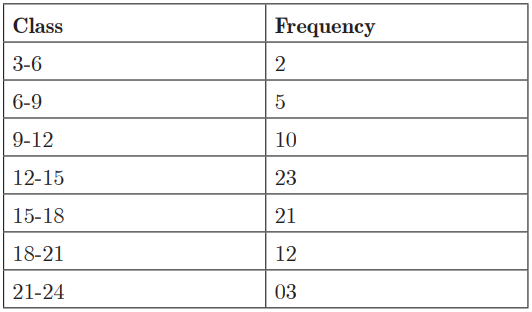Option A : 13.6

Option B : 15.6

Option C : 14.6

Option D : 16.6

Option C : 14.6

Statistics Class 10 MCQ Question No 45:

The median of a set of 9 distinct observations is 20.5. If each of the largest 4 observation of the set is increased by 2, then the median of the new set

Option A : is increased by 2

Option B : is decreased by 2

Option C : is two times the original median

Option D : remains the same

Option D : remains the same

Statistics Class 10 MCQ Question No 46:

If the mean and median of the data a , b and c are 50 and 35 respectively, where a < b < c. If c – a = 55, then find the value of (b – a).

Option A : 8

Option B : 7

Option C : 3

Option D : 5

Option D : 5

Statistics Class 10 MCQ Question No 47:

If the median of a series exceeds the mean by 3, find by what number the mode exceeds its mean?

Option A : 9

Option B : 6

Option C : 3

Option D : 1

Option A : 9

Statistics Class 10 MCQ Question No 48:

For following frequency distribution, find the median class.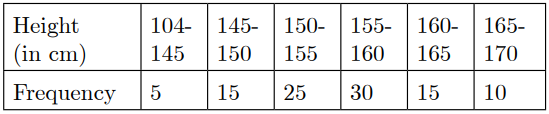Option A : 145-150

Option B : 155-160

Option C : 150-155

Option D : 160-165

Option C : 15

Statistics Class 10 MCQ Question No 49:

Find the mode of the following distribution.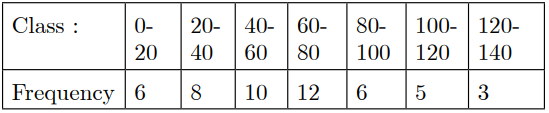Option A : 60

Option B : 65

Option C : 70

Option D : 75

Option B : 65

Statistics Class 10 MCQ Question No 50:

The mode of the following frequency distribution is 36. Find the missing frequency f.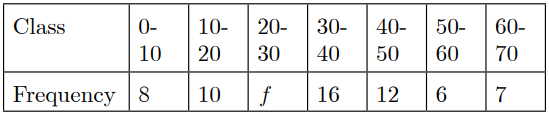Option A : 12

Option B : 16

Option C : 10

Option D : 9

Option C : 10

CBSE Class 10 Maths Chapterwise MCQs
1 : Real Numbers MCQ
| 1 to 10 | 11 to 20 | 21 to 30 | 31 to 40 | 41 to 50
2 : Polynomials MCQ
| 1 to 10 | 11 to 20 | 21 to 30 | 31 to 40 | 41 to 50
3 : Linear Equations MCQ
| 1 to 10 | 11 to 20 | 21 to 30 | 31 to 40 | 41 to 50
| 1 to 10 | 11 to 20 | 21 to 30 | 31 to 40 | 41 to 50
5 : Arithmetic Progression MCQ
| 1 to 10 | 11 to 20 | 21 to 30 | 31 to 40 | 41 to 50
6 : Triangles MCQ
| 1 to 10 | 11 to 20 | 21 to 30 | 31 to 40 | 41 to 50
7 : Coordinate Geometry MCQ
| 1 to 10 | 11 to 20 | 21 to 30 | 31 to 40 | 41 to 50
8 : Introduction to Trigonometry MCQ
| 1 to 10 | 11 to 20 | 21 to 30 | 31 to 40 | 41 to 50
9 : Application of Trigonometry MCQ
| 1 to 10 | 11 to 20 | 21 to 30 | 31 to 40 | 41 to 50
10 : Circles MCQ
| 1 to 10 | 11 to 20 | 21 to 30 | 31 to 40 | 41 to 50
11 : Constructions MCQ
| 1 to 10 | 11 to 20
12 : Area Related to Circles MCQ
| 1 to 10 | 11 to 20 | 21 to 30 | 31 to 40 | 41 to 50
13 : Surface Area & Volume MCQ
| 1 to 10 | 11 to 20 | 21 to 30 | 31 to 40 | 41 to 50
14 : Statistics MCQ
| 1 to 10 | 11 to 20 | 21 to 30 | 31 to 40 | 41 to 50
15 : Probability MCQ
| 1 to 10 | 11 to 20 | 21 to 30 | 31 to 40 | 41 to 50
16 : Previous Year Maths MCQs from CBSE Board Class 10
| 1 to 100
AMBiPi
error: Content is protected !!